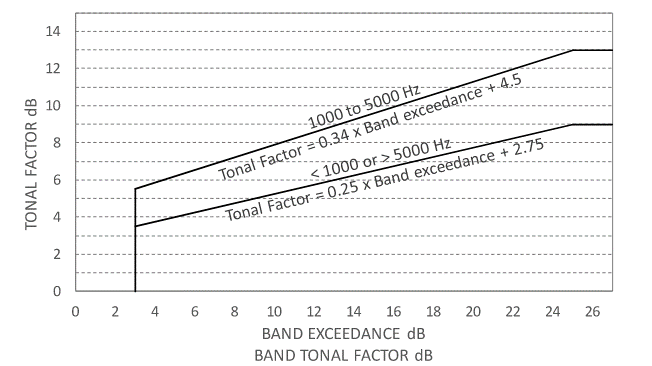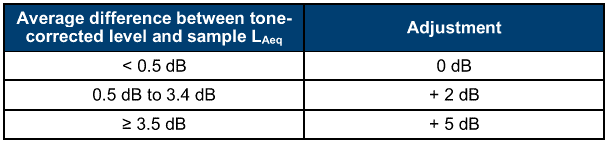### Strutt Help

VIC EPA 1826.4 Tonality    1/3,1/3LF

Strutt|Environmental Noise|Tonality|VIC EPA 1826.4 Tonality assesses the tonality of a 1/3 octave band sound pressure level spectrum by calculating the tone-corrected broadband level L_(TC), in accordance with Annex C of VIC EPA Noise Limit and Assessment Protocol, 1826.4.

The input spectrum should be unweighted.

L_(TC) is calculated as follows:

• For each one-third octave band, calculate the band exceedance BE_i for each 1/3 octave band i by taking the difference, rounded to one decimal place, between the A-weighted band level and the arithmetic average of the A-weighted levels of the two adjacent one-third octave bands as follows:

BE_i = L_(Ai) - (L_(Ai-1)+L_(Ai+1))/2 dB
• For one-third octave bands for which the band exceedance is greater than 3.0 dB, determine the tonal factor TF_i from Figure C1 of the protocol:• For one-third octave bands where the band level is 25 dB or more below the highest band level, the tonal factor TF_i is set to zero.
• Calculate a tone-corrected level L_(TC) across all one-third octave bands by combining the band levels of each band augmented by the relevant tonal factor, and rounding to one decimal place using:

L_(TC) = 10 * Log_(10)(\sum_(i=1)^j10^((L_(Ai)+TF_i)/10)) dB(A)

The user should calculate the L_(TC) for a selection of noise samples.

At least three samples must be made. Each sample must have a duration of at least one second and must represent the tonal character of the noise. The sum of the duration of the samples analysed must be at least 24 seconds.

The tonal correction can then be calculated as follows:

• For each sample, calculate the difference between the tone-corrected level and the uncorrected overall L_(Aeq) of the sample, rounded to one decimal place.

• Calculate the arithmetic average of these differences, rounded to one decimal place.

• Determine the tonal adjustment as per Table 7 in Annex C:References:

• Appendix C of VIC EPA Noise Limit and Assessment Protocol, 1826.4, May 2021